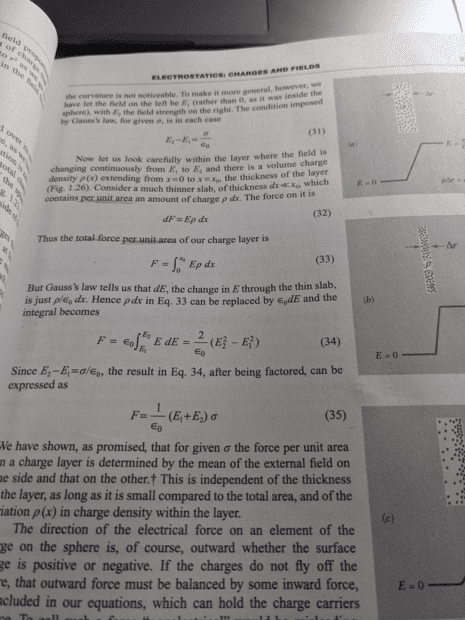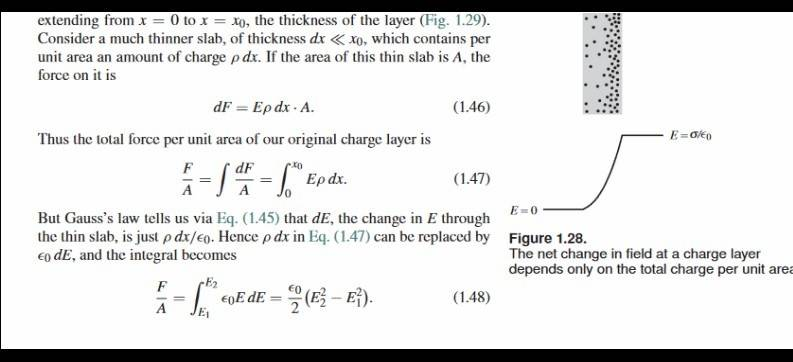# Why is this equation from Purcell's EM textbook correct?

Leo Liu
Homework Statement:
.
Relevant Equations:
.
I saw the following equation on page 31 in Purcell's EM textbook.
$$F=\epsilon_0\int_{E_1}^{E_2} E\, dE=\frac 2 {\epsilon_0} (E_2^2-E_1^2)$$
Here, F is the force on a unit area.
And then he claims that since ##E_2-E_1=\sigma/\epsilon_0##, the equation can be further simplified to
$$F=\frac 1 {\epsilon_0}(E_1+E_2)\sigma$$

However, I think the correct coefficient in the last part of first equation should be the inverse of what it is now (##\frac{\epsilon_0}{2}## instead), as only then can I obtain the second expression. I have no idea why the author wrote the equation this way. To give it a little bit of background, dE is the change in the electric field of a thin layer of a charged sphere, E1 is the the electric field inside, and E2 is the electric field outside. Could someone explain?

Last edited:

Homework Helper
Gold Member
yes, at least viewing from a purely mathematical view (integrating ##\int x dx=\frac{x^2}{2}## that should have been ##\frac{\epsilon_0}{2}##. Also from a dimensional analysis point of view ##\epsilon_0## just cannot be on the denominator, the units won't match then.

•Leo Liu and Steve4Physics
Homework Helper
Gold Member
2022 Award
Homework Statement:: .
Relevant Equations:: .

I saw the following equation on page 31 in Purcell's EM textbook.
$$F=\epsilon_0\int_{E_1}^{E_2} E\, dE=\frac 2 {\epsilon_0} (E_2^2-E_1^2)$$
The formula (1.48 in the book) is in fact
##\frac F A = … = \frac {\epsilon_0}{2}(E_2^2 – E_1^2)##

Leo Liu
The formula (1.48 in the book) is in fact
##\frac F A = … = \frac {\epsilon_0}{2}(E_2^2 – E_1^2)##Here is a picture of my book. This book is probably an older edition which is authorized to be printed in China by the original publisher.

Homework Helper
Gold Member
2022 Award
;View attachment 287228
Here is a picture of my book. This book is probably an older edition which is authorized to be printed in China by the original publisher.
I guess this is an error in earlier editions which has now been corrected. The 3rd edition (bottom of page 31) shows this:•Delta2 and Leo Liu